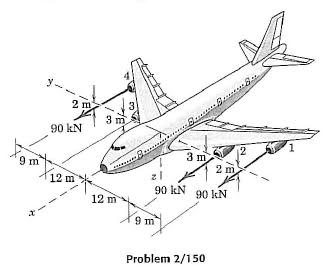# Statics - Resultant Force

## Homework StatementIf engine 3 suddenly fails, determine the resultant of the three remaining engine thrust vectors, each of which has a magnitude of 90kN. Specify the y and z-coordinates of the point through which the line of action of the resultant passes.

## The Attempt at a Solution

I did it but I have no idea whether my working is right or not.
The resultant force is 90kN x 3 = 270kN in the x direction.
To find the y and z coordinates, I looked for the resultant moment about point 0 which i chose to be at (0,0,0).
This is where I'm not sure. Why do the 2m and 3m arrows pointing out from the engines in the x direction matter? (Actually, they probably don't matter too much as they cancel out. But I have to show my working so it'll be good if someone can explain their significance)
I assumed they must have some effect so I multiplied 90kN by (2/3) for the two outer engines.
So, the moment due to the first force on the left is -21 x 90kN x (2/3) = -2520kN (in the z direction, ie pointing upwards)
The moment due to the inner engine on the right is 12 x 90kN = 1080kN (pointing downwards)
And the moment due to the rightmost engine is 21 x 90kN x (2/3) = 2520kN (pointing downwards)
The resultant moment is 1080kN (pointing downwards)

I know that the sum of moments about O is equal to the moment of the resultant force about O (Varignon's Theorem). So to find the y-coordinate of the point which the line of action of the resultant passes, I equated
1080kN = r x 270kN
r = 4
Therefore, the resultant force is 4m to the right of point 0.
The z coordinate should be zero.

Can someone tell me if I did it right? Any help would be much appreciated :)

## Answers and Replies

Yes this is correct!!

The 2m and 3m designations are the distance along the z-axis that the engines are away from the x-axis. These distances, coupled with the forces, will cause each engine to create moments, also.

edit: Oh, geez - I just noticed that this thread originated two years ago. Sorry...